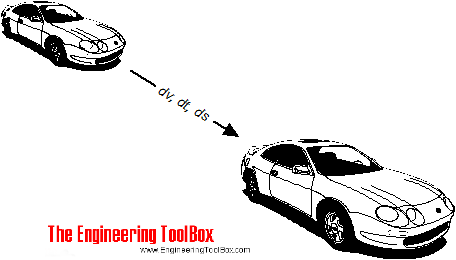Engineering ToolBox - Resources, Tools and Basic Information for Engineering and Design of Technical Applications!

# Acceleration Units Converter

## Converting between units of acceleration.Acceleration is the ratio change of velocity to change in time - and can be expressed as

a = dv / dt                          (1)

where

a = acceleration (m/s2, ft/s2)

dv = v1 - v0 = change in velocity (m/s, ft/s)

dt = t1 - t0 = time taken to change the velocity (s)

### Online Units Acceleration Calculator

The calculator below can be used to convert between some common acceleration units:

Value

m/s2

g

cm/s2

ft/s2

in/s2

km/hr2

ft/hr2

knot/s

miles/hr2

### Example - Acceleration of a Car

A car accelerates from 0 km/h (0 m/s) to 100 km/h (27.8 m/s) in 5 seconds. The acceleration can be calculated by using km/h as the units for velocity:

a = ((100 km/h) - (0 km/h)) (1000 m/km) (1/3600 h/s) / (5 s)

= 5.6 m/s2

The calculation can be simplified by using m/s as the units for velocity:

a = ((27.8 m/s) - (0 km/h)) / (5 s)

= 5.6 m/s2

## Related Topics

• Basics - The SI-system, unit converters, physical constants, drawing scales and more.
• Dynamics - Motion - velocity and acceleration, forces and torque.
• Mechanics - Forces, acceleration, displacement, vectors, motion, momentum, energy of objects and more.

## Engineering ToolBox - SketchUp Extension - Online 3D modeling!

Add standard and customized parametric components - like flange beams, lumbers, piping, stairs and more - to your Sketchup model with the Engineering ToolBox - SketchUp Extension - enabled for use with the amazing, fun and free SketchUp Make and SketchUp Pro .Add the Engineering ToolBox extension to your SketchUp from the SketchUp Pro Sketchup Extension Warehouse!

Translate

## Privacy

We don't collect information from our users. Only emails and answers are saved in our archive. Cookies are only used in the browser to improve user experience.

Some of our calculators and applications let you save application data to your local computer. These applications will - due to browser restrictions - send data between your browser and our server. We don't save this data.

## Citation

• Engineering ToolBox, (2008). Acceleration Units Converter. [online] Available at: https://www.engineeringtoolbox.com/acceleration-converter-d_1284.html [Accessed Day Mo. Year].

Modify access date.

. .

#### Scientific Online Calculator3 30